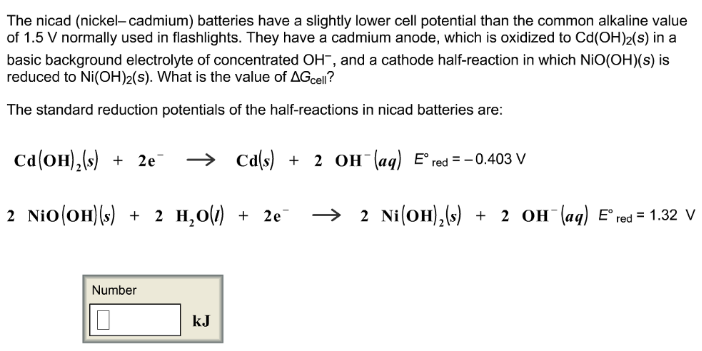# Problem: The nicad (nickel-cadmium) batteries have a slightly lower cell potential than the common alkaline value of 1.5 V normally used in flashlights. They have a cadmium anode, which is oxidized to Cd(OH)2(s) in a basic background electrolyte of concentrated OH-, and a cathode half-reaction in which NiO(OH)(s) is reduced to Ni(OH)2(s). What is the value of ΔG cell? The standard reduction potentials of the half-reactions in nicad batteries are: Cd (OH)2(s) + 2e- → Cd(s) + 2OH-(aq)          E°red = -0.403 V 2NiO(OH)(s) + 2H2O(l) + 2e- → 2Ni(OH)2(s) + 2OH-(aq)      E°red = 1.32 V

###### FREE Expert Solution
87% (407 ratings)###### Problem Details

The nicad (nickel-cadmium) batteries have a slightly lower cell potential than the common alkaline value of 1.5 V normally used in flashlights. They have a cadmium anode, which is oxidized to Cd(OH)2(s) in a basic background electrolyte of concentrated OH-, and a cathode half-reaction in which NiO(OH)(s) is reduced to Ni(OH)2(s). What is the value of ΔG cell

The standard reduction potentials of the half-reactions in nicad batteries are:

Cd (OH)2(s) + 2e- → Cd(s) + 2OH-(aq)          E°red = -0.403 V

2NiO(OH)(s) + 2H2O(l) + 2e- → 2Ni(OH)2(s) + 2OH-(aq)      E°red = 1.32 V